## 20.2. Creating and formatting basic odometer graphs

In order to use odometer graphs the extension module "`jpgraph_odo.php`" must be included in the script (in addition to the ordinary "`jpgraph.php`" code module).

The creation of Odometer graphs otherwise follows the traditional steps in the library of creating a graph and then adding one or several plots to the canvas.

1. Create a basic canvas graph as an instance of ```class OdoGraph```

2. Create an instance of one or several odometer plots (the dials) as instances of `class OdoPlot`, set up the scale and appearance and then add them to the graph canvas.

3. Send back the graph to the client with a call to `OdoGraph::Stroke()`

The following script shows the principles

 ```1 2 3 4 5 6 7 8 9 10 11 12 13 14 15 16 17 18 19 20 21 22 23 24 25 26 27 28 29 30 31 32 ``` ```title->Set(.......) \$graph->caption->Set(...) // Create one of more Odometer \$odo1 = new Odometer(); \$odo2 = new Odometer(); ... // Adjust odometer parameters, colors etc \$odo1->needle->Set(21); \$odo2->needle->Set(47); ... // Position the odometer plots vertically \$l = new LayoutVert( array(\$odo1, \$odo2, ...) ); // Add the odometers with the proper layout to the canvas \$graph->Add( \$l ); // If you only have a single odometer you may also write // \$graph->Add( \$odo1 ); // Send back the image to the client \$graph->Stroke(); ?>```

### 20.2.1. A basic odometer

The following script shows the simplest possible odometer using just default values

Example 20.1. A basic odometer (`odotutex00.php`)

 ```1 2 3 4 5 6 7 8 9 10 11 12 13 14 15 16 17 18 19 20 21 ``` ```needle->Set(30); // Add the odometer to the graph \$graph->Add(\$odo); // ... and finally stroke and stream the image back to the client \$graph->Stroke(); ?>```

Figure 20.2. A basic odometer `(odotutex00.php)`The size of the Odometer is determined automatically to fit the given image size as good as possible. Since the size of the odometer automatically adjust itself to allow for any odometer caption, graph titles or graph captions there is rarely need to manually override this automation.

However it is still possible to manually specify the absolute size (of the radius) in pixels or as a fraction of the image size.

To set a specific value top the Odometer radius the following method is used

• `OdoPlot::SetSize(\$aRadius)`

Since clas OdoGraph inherits all the basic graph features we can easily augment the graph in Figure 20.2 to, for example, have titles by adding the line

 ```1 ``` `\$graph->title->Set('A suitable graph title');`

In the following chapters we will go through all the options that exists to modify the size, shape and appearance of the graph.

### Tip

Remember that since Odometer inherits all the basic graph feature it will support functionality like image cache (see Section 5.6.)

### 20.2.2. Half and Full Odometers

Odometers comes in two fundamental shapes, full and half circle. The default if nothing else is specified is to create a half circle. The type of odometer is specified in the creation of the odometer.

The type is controlled by the two constants

• `ODO_FULL` Create a full circle odometer

• `ODO_HALF` Create a half circle odometer. This is also the default value.

As an example the following odometer plot instantiation will create a full odometer plot

 ```1 ``` `\$odo = new Odometer(ODO_FULL);`

and the following a half odometer plot

 ```1 ``` `\$odo = new Odometer(ODO_HALF);`

In ?? an example of a very basic full circle odometer is shown

Figure 20.3. A full circle odometer `(odotutex01.php)`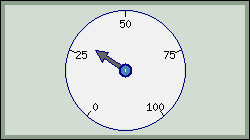### 20.2.3. Adding titles and captions

Remember that each graph can have a title, a subtitle and subsubtitle. In addition the Odmeter graphs offers the possibility to have a caption string. This is a text (much like the title) but is drawn at the bottom of the graph instead of at the top.

The following script shows how to add a tite, subtitle and caption to our previous example

Example 20.2. Adding titles and captions (`odotutex02.php`)

 ```1 2 3 4 5 6 7 8 9 10 11 12 13 14 15 16 17 18 19 20 21 22 23 24 25 26 27 28 29 30 ``` ```title->Set("Result for 2002"); \$graph->title->SetColor("white"); \$graph->title->SetFont(FF_ARIAL,FS_BOLD,14); \$graph->subtitle->Set("New York Office"); \$graph->subtitle->SetColor("white"); \$graph->caption->Set("Figure 1. Branch results."); \$graph->caption->SetColor("white"); // Now we need to create an odometer to add to the graph. // By default the scale will be 0 to 100 \$odo = new Odometer(); // Set display value for the odometer \$odo->needle->Set(30); // Add the odometer to the graph \$graph->Add(\$odo); // ... and finally stroke and stream the image back to the client \$graph->Stroke(); ?>```

Figure 20.4. Adding titles and captions `(odotutex02.php)`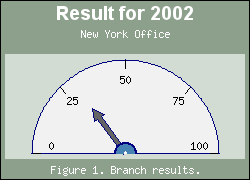As can be see from the figure the margins will automatically adjust to hold the specified titles and captions.

Remember that text strings can have multiple lines by separating lines with a '\n' character. The following example shows how to add a more descriptive caption to the graph

Example 20.3. Adding a multi line caption (`odotutex03.php`)

 ```1 2 3 4 5 6 7 8 9 10 11 12 13 14 15 16 17 18 19 20 21 22 23 24 25 26 27 28 29 30 ``` ```title->Set("Result for 2002"); \$graph->title->SetColor("white"); \$graph->title->SetFont(FF_ARIAL,FS_BOLD,14); \$graph->subtitle->Set("New York Office"); \$graph->subtitle->SetColor("white"); \$graph->caption->Set("Figure 1.This is a very, very\nlong text with multiples lines\nthat are added as a caption."); \$graph->caption->SetColor("white"); // Now we need to create an odometer to add to the graph. // By default the scale will be 0 to 100 \$odo = new Odometer(); // Set display value for the odometer \$odo->needle->Set(30); // Add the odometer to the graph \$graph->Add(\$odo); // ... and finally stroke and stream the image back to the client \$graph->Stroke(); ?>```

Figure 20.5. Adding a multi line caption `(odotutex03.php)`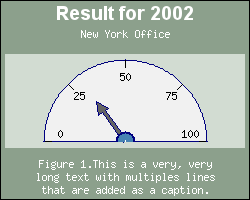Almost all areas in the graph have a customizable color. This means that for the Odometer graph itself it is possible to adjust

• Margin colors

• The plot area (the rectangular area where the plot(s) are added

• The border line colors

For example the following line changes the margin color of the graph

 ```1 2 3 4 5 ``` ```SetMarginColor("khaki:0.6"); \$graph->SetColor("khaki"); ?>```

For the odometer plot itself it is possible to adjust

• Fascia color

• Scale label color (and font)

• Frame color (and width)

• Indicator color (the color of the odometer "needle"

• Adding colored segment for scale areas

For example the following lines would change the fascia, indicator and scale label color

 ```1 2 3 4 5 ``` ```SetColor("white"); \$odo->scale->label->SetColor("blue"); \$odo->needle->SetFillColor("yellow"); ?>```

Figure 20.6 shows the effect of adding the two code snippets above to our previous example.

Figure 20.6. Changing colors `(odotutex04.php)`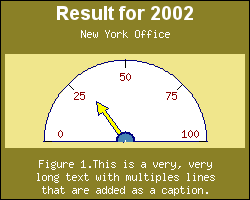### 20.2.5. Changing indicator needle style

The indicator is accessed through the "`\$needle`" property of the plot. The indicator needle for the odometer can have six basic shapes. which are set by the method

• `Needle::SetStyle(\$aStyle,\$aStyleOption=null)`

The style is defined by one of the following symbolic constants.

1. `NEEDLE_STYLE_SIMPLE`

2. `NEEDLE_STYLE_STRAIGHT`

3. `NEEDLE_STYLE_ENDARROW`, (default)

4. `NEEDLE_STYLE_SMALL_TRIANGLE`

5. `NEEDLE_STYLE_MEDIUM_TRIANGLE`

6. `NEEDLE_STYLE_LARGE_TRIANGLE`

7. `NEEDLE_STYLE_HUGE_TRIANGLE`

The following line would therefore create a plot with a "simple" indicator.

 ```1 ``` `\$odo->needle->SetStyle(NEEDLE_STYLE_SIMPLE);`

The different styles are illustrated in Figure 20.7

Figure 20.7. Possible shapes of the odometer indicator `(odotutex06.php)`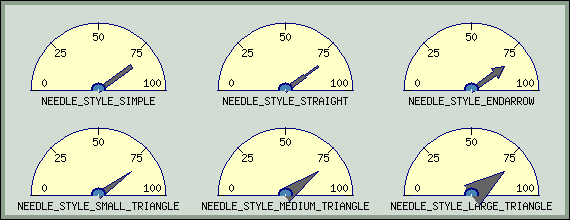For the needle style "`NEEDLE_STYLE_ENDARROW`" it is also possible to adjust the ending arrow. This is done by specifying the particular end arrow size as the second parameter to `SetStyle()`. , the `\$aStyleOption` parameter.

To simplify the arrow style setting a number of predefined sizes are available. When choosing an arrowhead the width and length are specified in three different steps, small , medium and large.

The available end arrow styles are listed in ?? below

Table 20.1. Available arrow size

Symbolic nameDescription
`NEEDLE_ARROW_SS`Small width, small length
`NEEDLE_ARROW_SM`Small width, small length
`NEEDLE_ARROW_SL`Small width, large length
`NEEDLE_ARROW_MS`Medium width, small length
`NEEDLE_ARROW_MM`Medium width, small length
`NEEDLE_ARROW_ML`Medium width, large length
`NEEDLE_ARROW_LS`Large width, small length
`NEEDLE_ARROW_LM`Large width, medium length
`NEEDLE_ARROW_LL`Large width, large length

The following code specifies a the largest available arrowhead

 ```1 ``` `\$odo->needle->SetStyle(NEEDLE_STYLE_ARROWHEAD, NEEDLE_ARROW_LL);`

An illustration of the various arrow heads are given in Figure 20.8

Figure 20.8. Illustration of various sizes of arrow heads `(odotutex07.php)`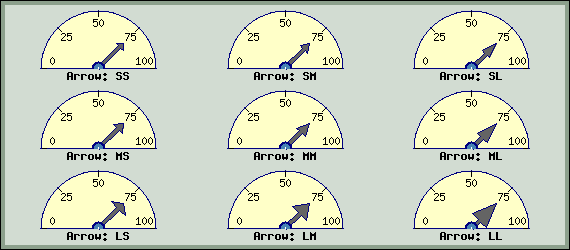The basic length and weight of the needle is specified with the methods

• `Needle::SetLength(\$aLength)`

• `Needle::SetWeight(\$aWeight)`

The needle size is specified as fractions of the radius. So for example the following line wold create a needle halfway into the plot area

 ```1 ``` `\$odo->needle->SetLength(0.5);`

The default size for a needles is 60% of the radius.

It is possible to add drop shadows to both the overall graph s well as the indicator needle. To add a drop shadow to the overall graph the method is as usual

• `OdoGraph::SetShadow(\$aShadow=true,\$aColor="gray@0.5",\$aDx=4,\$aDy=4)`

and to add a drop shadow to the indicator needle the method

• `OdoNeedle::SetShadow(\$aShadow=true,\$aColor="gray@0.5",\$aDx=4,\$aDy=4)`

must be used.

The following two lines add both types of drop shadows to an odometer graph with the result shown in ??

 ```1 2 3 4 5 ``` ```SetShadow(); ... \$odo->needle->SetShadow(); ?>```

Figure 20.9. Adding drop shadows `(odotutex08.php)`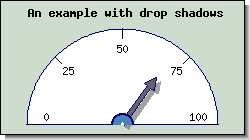### 20.2.7. Changing sizes and margins

The size of the odometer is automatically adjusted to fit the available space in the image after margins for titles and captions have been taken into account. This means that normally it is not necessary to adjust the margins manually.

The odometer plot can be adjusted with the method

• `OdoPlot::SetSiz<e(\$aSize)`

Each odometer also have a margin surrounding it. This is the amount of "space" around each individual odometer. The amount of space is adjusted by the method

OdoPlot::SetMargin(\$aMargin)

If we take the example in Figure 20.8 and increase the individual margin around all odometers they will become smaller (if we keep the original image size) and the result is shown in Figure 20.10

Figure 20.10. Increasing the individual margins around the plots `(odotutex09.php)`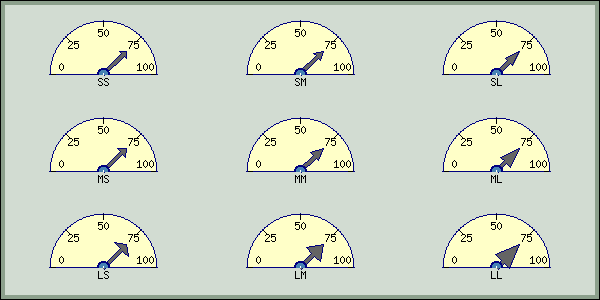### 20.2.8. Adding color indications in the scale

An odometer usually have some colored markings to indicate safe and dangerous values. The library allows the addition of any number of colored scale areas with one or several calls to the method

• `OdoPlot::AddIndication(\$aMin,\$aMax,\$aColor)`

This method allows the specification of a min and max value of the scale which will be given the background color specified as the third parameter.

The example in Figure 20.11 adds three different scale area with 6 different scale areas

Figure 20.11. Adding colored scale indicators `(odotutex10.php)`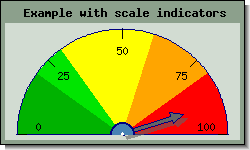### 20.2.9. Changing size of the non-colored center area

In Figure 20.11 it can be seen that by default the scale color indication goes all the way down to the base of the needle. It is however possible to adjust this to the colored area stops a bit above the base of the needle. The non colored area is adjusted with a call to the method

• `OdoPlot::SetCenterAreaWidth(\$aWidth)`

The width is specified as a fraction of the radius of the plot.

The following example in Figure 20.12set the size of this base area to be 45% of the plot radius.

Figure 20.12. Adjusting the non-colored center area `(odotutex11.php)`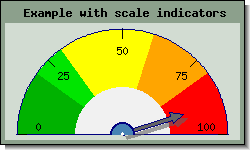### 20.2.10. Using multiple indicator needles in one odometer

In all the previous example the odometer plots have all had a single indicator. It is however possible to add up to four indicators to each plot. This could for example be sued to show the current value and a sliding 3-day average.

The extra needles are accessed through the properties

• `OdoPlot::needle2`

• `OdoPlot::needle3`

• `OdoPlot::needle4`

In order to enable one of these extra needles the method OdoNeedle::Show() has to be called. The value of the indicator can then be set as the following example shows.

 ```1 2 ``` ```\$odo->needle2->Show(); \$odo->needle2->Set(44);```

By default all the needles will have the same color so the color should be manually changed to be able to differentiate among multiple needles. The example in Figure 20.13 shows an example of this where we also have made the second indicator a bit shorter to further make it easier to read.

Figure 20.13. Adding a second scale indicator `(odotutex12.php)`### 20.2.11. Adding an odometer legend (label)

Most types of odometers have a legend in the middle of the meter usually indicating what the scale shows. The odometer have a specific text property to add text in that position.

• `OdoPlot::label`

The following code adds the label "mBar" as the scale indicator and also adjusts the default font

 ```1 2 ``` ```\$odo->label->Set("mBar"); \$odo->label->SetFont(FF_FONT2,FS_BOLD);```

The result of this cane be seen in Figure 20.14

Figure 20.14. Adding a scale legend by using the label property `(odotutex13.php)`### 20.2.12. Adjusting the width and color of the odometer frame (border)

The border of the odometer plot can be adjusted with a call to

• `OdoPlot::SetBorder(\$aColor,\$aWidth=1)`

The following example shows the effect of making the odometer plot border thicker

 ```1 ``` `"=odotutex08.1|Adding a thicker border around the odometer plot"`